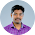# MATLAB Program for Formation of Bus Admittance Matrices | EE1404 – Power System Simulation Laboratory

AIM:
To determine the admittance matrices for the given power system network.

SOFTWARE REQUIRED:
MATLAB

THEORY:
Bus admittance is often used in power system studies. In most of the power system studies
it is required to form y- bus matrix of the system by considering certain power system parameters
depending upon the type of analysis.
Y-bus may be formed by inspection method only if there is no mutual coupling between the
lines. Every transmission line should be represented by
p- equivalent. Shunt impedances are
added to diagonal element corresponding to the buses at which these are connected. The off
diagonal elements are unaffected. The equivalent circuit of Tap changing transformers is included
while forming Y-bus matrix.

FORMATION OF Y-BUS MATRIX:
Generalised Y-bus = yii ……….. yid
ydi ……… ydd

PROCEDURE:
1. Enter the command window of the MATLAB.
2. Create a new M – file by selecting File - New – M – File
3. Type and save the program in the editor window.
4. Execute the program by either pressing Tools – Run.
5. View the results.

EXERCISE:
(i) Determine the Y bus matrix and Z bus matrix for the power system network shown in fig.
(ii) Check the results obtained in using MATLAB.

PROGRAM:
z = [0 1 0 1.0
0 2 0 0.8
1 2 0 0.4
1 3 0 0.2
2 3 0 0.2
3 4 0 0.08];
Y = ybus(z)

RESULT:
Thus the the admittance matrices for the given power system network determined.
Previous
Next Post »